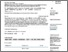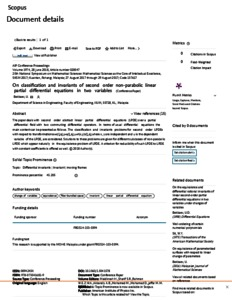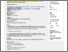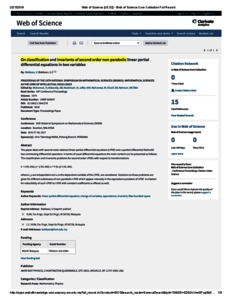# On classification and invariants of second order non-parabolic linear partial differential equations in two variables

Bekbaev, Ural (2018) On classification and invariants of second order non-parabolic linear partial differential equations in two variables. In: 25th National Symposium on Mathematical Sciences (SKSM25), 27th-29th August 2017, Kuantan, Pahang.PDF - Published Version
Restricted to Registered users onlyPreview
PDF (SCOPUS) - Supplemental MaterialPreview
PDF (WOS) - Supplemental Material

## Abstract

The paper deals with second order abstract linear partial differential equations (LPDE) over a partial differential field with two commuting differential operators. In terms of usual differential equations the main content can be presented as follows. The classification and invariants problems for second order LPDEs with respect to transformations x = x(x ;h); y = y(x ;h); u = h(x;y)v(x ;h); where x;y are independent and u is the dependent variable of the LPDE, are considered. Solutions to these problems are given for different subclasses of non-parabolic LPDE which appear naturally in the equivalence problem of LPDE. A criterion for reducibility of such LPDE to LPDE with constant coefficients is offered as well.

Item Type: Conference or Workshop Item (Plenary Papers) 6830/69402 linear partial differential equation; change of variables; equivalence; invariant; fiber bundled space Q Science > Q Science (General)Q Science > QA MathematicsQ Science > QA Mathematics > QA300 Analysis Kulliyyah of EngineeringKulliyyah of Engineering > Department of Science Associate Prof. Ural Bekbaev 23 Jan 2019 10:08 21 Feb 2019 12:11 http://irep.iium.edu.my/id/eprint/69402View Item